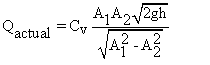# Flow through Venturi meter

Theory

Venturimeter is the most widely used device to measure the discharge through the pipe. A venturi is a converging-Diverging nozzle of circular cross-section.

The principle of venturimeter is that when a fluid flows through the venturimeter,   it accelerates in the convergent section and decelerates in the divergent section, resulting in a drop in the static pressure followed by a pressure recovery in the flow direction. By measuring the difference in the pressures at an axial station upstream of the convergent section and at the throat, the volumetric flow rate can be estimated.

The flow through the venturimeter and hence through the pipe is given by

###where

Q actual = Actual discharge in m 3 /s

C v =Discharge co-efficient of a venturimeter

A1 =area at the inlet side in m 2

A2 =area at the throat side in m 2

h =Differential pressure head of liquid in m

g =Acceleration due to gravity (9.81 m/s 2 )